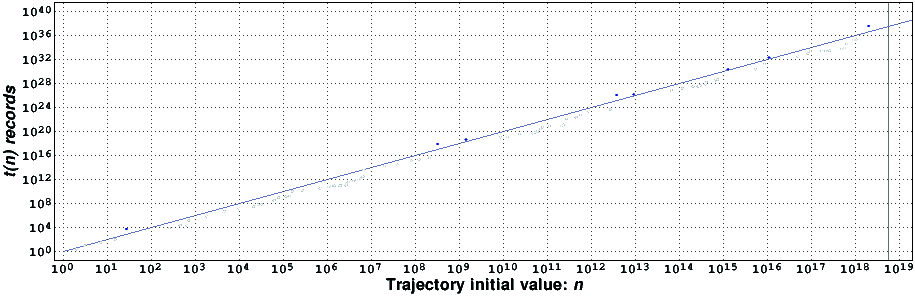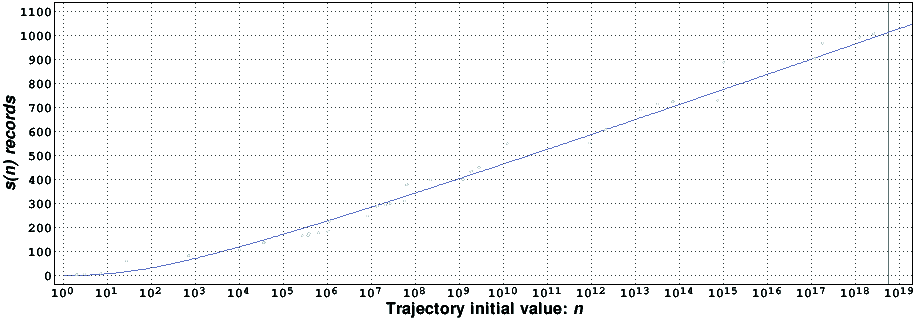# Computational verification of the 3x+1 conjecture

## Introduction

Let x be an integer. Let the function T(x) be equal to (3x+1)/2 if x is odd and equal to x/2 if x is even. The 3x+1 conjecture , [2, problem E16] asserts that starting from any positive integer n the repeated iteration of T(x) eventually produces the integer 1, after which the iterates will alternate between the integers 1 and 2.

Let n>1 be an integer. The trajectory of n is the sequence { n, T(n), T(T(n)), ..., T^(k)(n), ... }, where T^(k)(n) denotes the k-th iterate of T(x) for an initial value of n. The stopping time of n, denoted by s(n), is the least positive integer k for which T^(k)(n)<n, if it exists, or infinity, otherwise. The maximum excursion of n, denoted by t(n), is the maximum value of the trajectory of n, if that number exists, or infinity, otherwise. In the first case m(n) denotes the smallest iterate number where the maximum occurred. Furthermore, n is a maximum excursion record-holder if t(m)<t(n) for all integers m belonging to the interval 1<m<n, and n is a stopping time record-holder if s(m)<s(n) for all integers m belonging to the interval 1<m<n.

## News

September 21, 2004: 2^58 reached. Eighty two maximum excursion record-holders and thirty three stopping time record-holders.
December 16, 2004: 2·2^58 reached. Two new maximum excursion record-holders.
February 23, 2005: 3·2^58 reached. One new maximum excursion record-holder.
April 8, 2005: 4·2^58 reached. Two new maximum excursion record-holders.
May 25, 2005: 5·2^58 reached. One new stopping time record-holder.
August 2, 2005: 6·2^58 reached.
October 26, 2005: 7·2^58 reached. One new maximum excursion record-holder.
February 7, 2006: 8·2^58 reached.
March 29, 2006: 9·2^58 reached.
May 26, 2006: 10·2^58 reached. One new stopping time record-holder (the first above 1000).
October 28, 2006: 11·2^58 reached.
December 12, 2006: 12·2^58 reached.
February 1, 2007: 13·2^58 reached.
May 23, 2007: 14·2^58 reached.
November 9, 2007: 15·2^58 reached.
January 4, 2008: 16·2^58 reached.
February 21, 2008: 17·2^58 reached.
June 1, 2008: 18·2^58 reached.
September 4, 2008: 19·2^58 reached.
January 18, 2009: 20·2^58 reached. All computations stopped (they will be restarted, perhaps in 2016, using FPGAs).

## Computational results

In order to test the 3x+1 conjecture, in 1996 we wrote a computer program (in the programming language C), which computed the trajectories of all initial values of n smaller that a given limit and having a stopping time known to be larger than 40 . Each run of that program tested an interval of 2^50 integers. On an otherwise idle 266MHz DEC Alpha computer, the original program tested, on the average, an interval of approximately 317 million integers each second. Elementary improvements of the verification algorithm, the most important of which was suggested by Eric Roosendaal, raised this number to around 370 million. This program was run between August 1996 and April 2000 on two 133MHz and two 266MHz DEC Alpha workstations. We stopped it when 100·2^50 was reached. Around 14.4 CPU years were used in this verification.

In the Spring of 2004 we devised an improved algorithm  to test the 3x+1 conjecture, about three times faster than our previous one. Each pass of the new algorithm tests (and double-checks) an interval of 2^58 integers. The entire computation can be split among several computers. We restarted our verification efforts in June 2004, and stopped them in January 2009. We have verified the 3x+1 conjecture up to

20·2^58 = 5764607523034234880 > 5.764·10^18.

Around 81.1 CPU years were used in this verification. Since no counter-example was found, the 3x+1 conjecture is probably true for all positive integers not larger than this verification limit. (The word probably in the previous sentence is to guard against machine or algorithm errors; we had extreme care with the latter but cannot control the former. The machines where the program was run are reasonably reliable, and since we double check all computations we have great confidence that our results are correct.) Eric Roosendaal and collaborators have independently verified the 3x+1 conjecture up to 2^60 (May 2011).

Our program also determines all maximum excursion and stopping time record-holders occurring inside the verification interval. In the following figure we present the maximum excursion record-holders found [3KiB, compressed with gzip] in the verification effort.It is remarkable that the t(n) records are always close to n^2. Only a few are actually larger than n^2 (the blue dots). In fact, it appears that t(n)<n^2 f(n), where f(n) is either a constant or a very slowly increasing function of n. This is in agreement with the stochastic results reported in . In the following figure we present the stopping time record-holders found [1KiB, compressed with gzip] in the verification effort.Contrary to the t(n) records, the s(n) records appear to grow in a more erratic way. Nonetheless, they appear to be approximately proportional to the logarithm of n. Using the ideas of Terras  it is possible to estimate when a given stopping time will occur for the first time (the x coordinate of the blue curve is the inverse of the probability that the coefficient stopping time is not smaller that the corresponding y coordinate).

All record-holders between 100·2^50 and 309·2^50 were first discovered by Eric Roosendaal and his collaborators.

## References

  Jeffrey C. Lagarias, The 3x+1 problem and its generalizations, The American Mathematical Monthly, vol. 92, no. 1, pp. 3-23, 1985.  Richard K. Guy, Unsolved problems in number theory, third edition, Springer-Verlag, 2004.  Tomás Oliveira e Silva, Maximum excursion and stopping time record-holders for the 3x+1 problem: computational results, Mathematics of Computation, vol. 68, no. 225, pp. 371-384, 1999.  Tomás Oliveira e Silva, Empirical Verification of the 3x+1 and Related Conjectures. In The Ultimate Challenge: The 3x+1 Problem (book edited by Jeffrey C. Lagarias), pp. 189-207, American Mathematical Society, 2010.  J. C. Lagarias and A. Weiss, The 3x+1 problem: two stochastic models, The Annals of Applied Probability, vol. 2, no. 1, pp. 229-261, 1992.  Riho Terras, A stopping time problem on the positive integers, Acta Arithmetica, vol. XXX, pp. 241-252, 1976 (see also vol. XXXV, pp. 101-102, 1979).